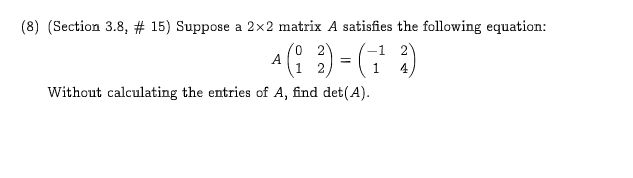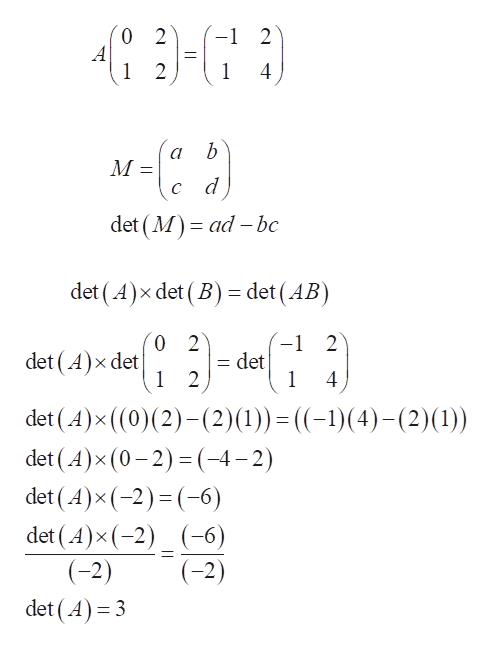# (8) (Section 3.8, # 15) Suppose a 2x2 matrix A satisfies the following equation:0 2A114Without calculating the entries of A, find det(A)

Question

Please the attachment for the question. Thankshelp_outlineImage Transcriptionclose(8) (Section 3.8, # 15) Suppose a 2x2 matrix A satisfies the following equation: 0 2 A 1 1 4 Without calculating the entries of A, find det(A) fullscreen
check_circle

Step 1

Given equation is:

Determinant of a matrix is given by:

According to the properties of determinant...help_outlineImage Transcriptionclose0 2 A 1 2 2 1 4 b а М c d det (M ad bc det (A) x det (B) = det (AB 0 2 det (A) x det 1 2 1 2 det 1 det (A)x ((0)(2)(2)(1)) det (A)x (0-2)(-4-2) det (A)x (-2) (-6) det (A)x (-2) (-6) (-2) ((-1)(4)-(2) (1)) (-2) det (A)3 fullscreen

### Want to see the full answer?

See Solution

#### Want to see this answer and more?

Solutions are written by subject experts who are available 24/7. Questions are typically answered within 1 hour.*

See Solution
*Response times may vary by subject and question.
Tagged in

### Matrices